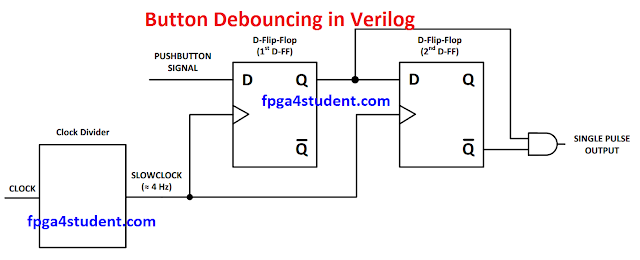# Verilog code for debouncing buttons on FPGA

## This post is to present a simple debouncing Verilog code for buttons on FPGA.

### Mechanical switches/ buttons cause the unpredictable bounce in the signal when toggled. There are various ways to implement debouncing circuits for buttons on FPGA. In this project, a simple debouncing circuit is implemented in Verilog to generate only a single pulse when pressing a button on FPGA.#### Debouncing Circuit for buttons on FPGA

As shown in the figure below, when a button on FPGA is pressed and released, there are many unexpected up-and-down bounces in push button signal. The debouncing circuit only generates a single pulse with a period of the slow clock without bouncing as we expected.### Verilog code for button debouncing on FPGA (extra FF to avoid metastability for the 1st FF):

```//fpga4student.com
// FPGA projects, Verilog projects, VHDL projects
// Verilog code for button debouncing on FPGA
// debouncing module
module debounce(input pb_1,clk,output pb_out);
wire slow_clk;
wire Q1,Q2,Q2_bar,Q0;
clock_div u1(clk,slow_clk);```
```my_dff d0(slow_clk, pb_1,Q0 );
my_dff d1(slow_clk, Q0,Q1 );
my_dff d2(slow_clk, Q1,Q2 );
assign Q2_bar = ~Q2;
assign pb_out = Q1 & Q2_bar;
endmodule
// Slow clock for debouncing
module clock_div(input Clk_100M, output reg slow_clk

);
reg [26:0]counter=0;
always @(posedge Clk_100M)
begin
counter <= (counter>=249999)?0:counter+1;
slow_clk <= (counter < 125000)?1'b0:1'b1;
end
endmodule
// D-flip-flop for debouncing module
module my_dff(input DFF_CLOCK, D, output reg Q);

always @ (posedge DFF_CLOCK) begin
Q <= D;
end

endmodule
```

#### Simulation waveform for button debouncing (2FFs):As shown in the waveform, only a single pulse is generated when a button is pressed and released as expected.
It is noted that this code is about to create another clock domain in your design, so you have to take care of the multi-clock domain issues while designing such as interfacing signals between different clock domains (synchronizers needed, etc.). Instead of creating another slow clock in the design, we can generate the clock enable signal to drive the debouncing flip-flops.

#### The better Verilog code for debouncing buttons on FPGA without creating another clock domain:

```//fpga4student.com: FPGA projects, Verilog projects, VHDL projects
// Verilog code for button debouncing on FPGA
// debouncing module without creating another clock domain
// by using clock enable signal
module debounce_better_version(input pb_1,clk,output pb_out);
wire slow_clk_en;
wire Q1,Q2,Q2_bar,Q0;
clock_enable u1(clk,slow_clk_en);```
```my_dff_en d0(clk,slow_clk_en,pb_1,Q0);
my_dff_en d1(clk,slow_clk_en,Q0,Q1);
my_dff_en d2(clk,slow_clk_en,Q1,Q2);
assign Q2_bar = ~Q2;
assign pb_out = Q1 & Q2_bar;
endmodule
// Slow clock enable for debouncing button
module clock_enable(input Clk_100M,output slow_clk_en);
reg [26:0]counter=0;
always @(posedge Clk_100M)
begin
counter <= (counter>=249999)?0:counter+1;
end```
```    assign slow_clk_en = (counter == 249999)?1'b1:1'b0;
endmodule
// D-flip-flop with clock enable signal for debouncing module
module my_dff_en(input DFF_CLOCK, clock_enable,D, output reg Q=0);
always @ (posedge DFF_CLOCK) begin
if(clock_enable==1)
Q <= D;
end
endmodule
```

#### Testbench Verilog code for debouncing buttons:

````timescale 1ns / 1ps
// testbench verilog code for debouncing button without creating another clock
module tb_button;
// Inputs
reg pb_1;
reg clk;
// Outputs
wire pb_out;
// Instantiate the debouncing Verilog code
debounce_better_version uut (
.pb_1(pb_1),
.clk(clk),
.pb_out(pb_out)
);
initial begin
clk = 0;
forever #10 clk = ~clk;
end
initial begin
pb_1 = 0;
#10;
pb_1=1;
#20;
pb_1 = 0;
#10;
pb_1=1;
#30;
pb_1 = 0;
#10;
pb_1=1;
#40;
pb_1 = 0;
#10;
pb_1=1;
#30;
pb_1 = 0;
#10;
pb_1=1;
#1000;
pb_1 = 0;
#10;
pb_1=1;
#20;
pb_1 = 0;
#10;
pb_1=1;
#30;
pb_1 = 0;
#10;
pb_1=1;
#40;
pb_1 = 0;
end

endmodule
```
Simulation Waveform of the Verilog code for debouncing buttons:As shown in the simulation waveform, only a pulse with a period of the slow clock enable signal is generated when a button is pressed, held enough, and released as expected. Note that the divisor of 9 is used for faster simulation. If you want a single pulse with a period of the clock input to be generated, simply modify the output assignment "assign pb_out = Q1 & Q2_bar & slow_clk_en;".
VHDL code for debouncing buttons on FPGA
Recommended Verilog projects:

1.can anyone publish the code for 8bit parity checker ?

1.Noted. Thanks.

2.Did not get the same waveform for the second version. Counter never reaches 249999 causing the clock enable to be always 0, causing the DFFs always outputting zero.

1.For simulation, a smaller value of divisor in clock enable was use for faster simulations.

3.I have a question on the improved version: the slow_clk_en is only active when the button is pressed, how will trigger D1 return to zero? I mean, will it fail to register the second full button press? Or am I missing something?

1.Yes. Thanks. You are right. There was a mistake. The code now has been updated and verified both in simulation and on FPGA.